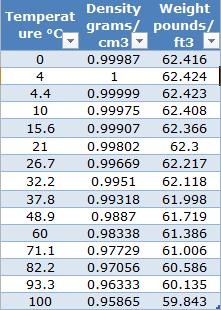# Unfortunately,

Excel does not provide a function for linear interpolations. If your data table has a low granularity (you have only units, not sub – units), and you need precise results, you have to create your own linear interpolation formula. You will find in this article an excel formula, and a User Defined Function (UDF) for Linear Interpolation in Excel.

Granularity refers to the level of detail of the data; a high level of detail means a low level of granularity, a low level of detail means a high level of granularity.

Basically, the Excel formula is simple: =_X1+(_X2 – _X1)*(Y – _Y1)/(_Y2 – _Y1)Linear Interpolation[/captionhttps

This formula can be written in a more familiar style, the relationship between the known points (X1,Y1) and (X2,Y2) and unknown location of X, Y is:For the below data table, the problem is that finding the _X1, _X2 and _Y1, _Y2 values requires 4 different formulas, written in 4 defined names. I will not dive into details, but you can download the sample workbook, to see how it works, you will find a lot of details and explanations for each part of the formula.

[caption id="attachment_154" align="aligncenter" width="214"]Data Table

The UDF I developed for Linear Interpolations, makes life easier 🙂

Here is the VBA code:

```Option Explicit
Function Interpolate(Y As Double, Tbl As Range, _
RdColData As Long, Optional VerticalTable As Boolean = True)
Dim HorizontalTable As Label
'Catalin Bombea - Iasi, 2013

'first argument - TargetVal As Double is the reference value

'second argument - Tbl As Range,, is the data range;

'the third argument - RdColData As Integer, represents the column number of the data range, from this column the function will extract values.
'(must be set to Long instead of integer, for large tables of data)
'If you want to return results from second column, set this argument to 2.
'in horizontal tables, if you want to return data from 3'rd row, set this argument to 3.
'This argument can be 1, but because the function will always look for the reference value in first column for vertical data tables,
'or in first row for horizontal data tables, it will return the same data type as the reference value

'last argument-Optional VerticalTable As Boolean = True : "TRUE or FALSE. We should use TRUE (this argument is optional, the default value is TRUE)
'for vertical tables, if we have data in 2 or more columns;
'If the table is horizontal, (with data in 2 or more rows, but several columns of data), FALSE value must be used.
'IF FALSE is used, then the numeric argument will represent the ROW number to return values from, instead of COLUMN number).
'IMPORTANT:
'If this argument is TRUE, the function will look in the first COLUMN of the data range to find the reference value,
'and it will return values from one of the next columns, based on the value of the third argument; if this argument is FALSE,
'the function will search the reference value in the first ROW of the data table, and it will return values from one of the next rows,
'based on the value of the third argument."

Application.Volatile
Application.ScreenUpdating = False

Dim Y1 As Double, Y2 As Double, X1 As Double, X2 As Double, i As Long

If VerticalTable = False Then GoTo HorizontalTable
'when the loop ends, the next item is Y2, the closest higher value from data table
For i = 1 To Tbl.Rows.Count
If IsNumeric(Tbl.Cells(i, 1)) And Tbl.Cells(i, 1) > Y Then
Exit For
End If
Next i

Y1 = Tbl.Cells(i - 1, 1)
Y2 = Tbl.Cells(i, 1)
X1 = Tbl.Cells(i - 1, RdColData)
X2 = Tbl.Cells(i, RdColData)

Interpolate = X1 + (X2 - X1) * (Y - Y1) / (Y2 - Y1)
Application.ScreenUpdating = True
Exit Function

HorizontalTable:

For i = 1 To Tbl.Columns.Count
'when the loop ends, the next item is Y2, the closest higher value from data table
If IsNumeric(Tbl.Cells(1, i)) And Tbl.Cells(1, i) > Y Then
Exit For
End If
Next i

Y1 = Tbl.Cells(1, i - 1)
Y2 = Tbl.Cells(1, i)
X1 = Tbl.Cells(RdColData, i - 1)
X2 = Tbl.Cells(RdColData, i)

Interpolate = X1 + (X2 - X1) * (Y - Y1) / (Y2 - Y1)
Application.ScreenUpdating = True
End Function
```

The function is flexible, you can use it for both vertical or horizontal data tables, changing the last argument to TRUE or FALSE. All arguments are explained inside the code, and in the sample workbook, where you will find examples for both situations.

For the data table presented above, the UDF will look like this in a worksheet formula:

#### =Interpolate(Y, DataTable, 2, TRUE)

Note that the 4th argument for data table layout is optional, and by default, the value is TRUE (for Vertical data tables), you can omit this argument for vertical tables. If your data table is presented in an horizontal layout, the 4th argument must not be omitted, and the value should be FALSE:

#### =Interpolate(Y, DataTable, 2, False)

If this function makes you happy, let me know 🙂

This site uses Akismet to reduce spam. Learn how your comment data is processed.

The Excel Learning Journey that I propose for you, is structured in such a way as to offer you the most practical way to learn Excel, free.